# Examples for researchHigeo

"Now, I'll tell how to do research!"Pyon

"Really? I think it's so hard."Penta

"Me too."Higeo

"Non, non. It's easier than you think. You don't have to solve problems. You only have to change conditions of problems. I know that some students who usually get bad marks in math can change math problems very well"

We will introduce some examples to show how to do research and discover things. The examples are based on the research done by our group.

There are three basic steps in our method of research.

1. 1. Find a proper problem

2. 2. Change the problem instead of solving it. It may be good to solve it, if the problem is easy.

3. 3. Analyze the problem. You can use a mathematical software.

Here, we don't tell how to use mathematical softwares.

## Question

There are one red card and five white cards in box. Two players take turns and draw card from the box. If one of players draw the red card, this player loses. Once a card is drawn, it will not be returned to the box.

(We originally studied this problem as a Russian roulette.)### How to change some parts of problem.

The parts of the problem that can be changes are colored in red or blue.

There are one red card and five white cards in box. Two players take turns and draw card from the box. If one of players draw the red card, this player loses. Once a card is drawn, it will not be returned to the box.

If we change the parts in red color, the condition on numbers are changed.

There are one red card and five white cards in box. Two players take turns and draw card from the box. If one of players draw the red card, this player loses . Once a card is drawn, it will not be returned to the box.

If we change the parts in blue color, we change the rule of the game.

### Modifications made by our group.

We have made a lot of research results using this method of changing the original problems.

We are going to show how we have changed problems to make new discoveries. These researches eventually have reached the level of professional mathematicians, since many of our papers have been accepted by professional math journals.

In 1992, the members of research team at the time changed the original problem as the followings.

There are n red card and n - m white cards in box. Two player draw card alternately. If a player draw the red card, the player loses. The card don't back into the box.

When m red cards and n- m white cards are in a box, let f(n,m) be the probability of the first player's losing in the game.

By changing the values of n and m, we made a triangle like this.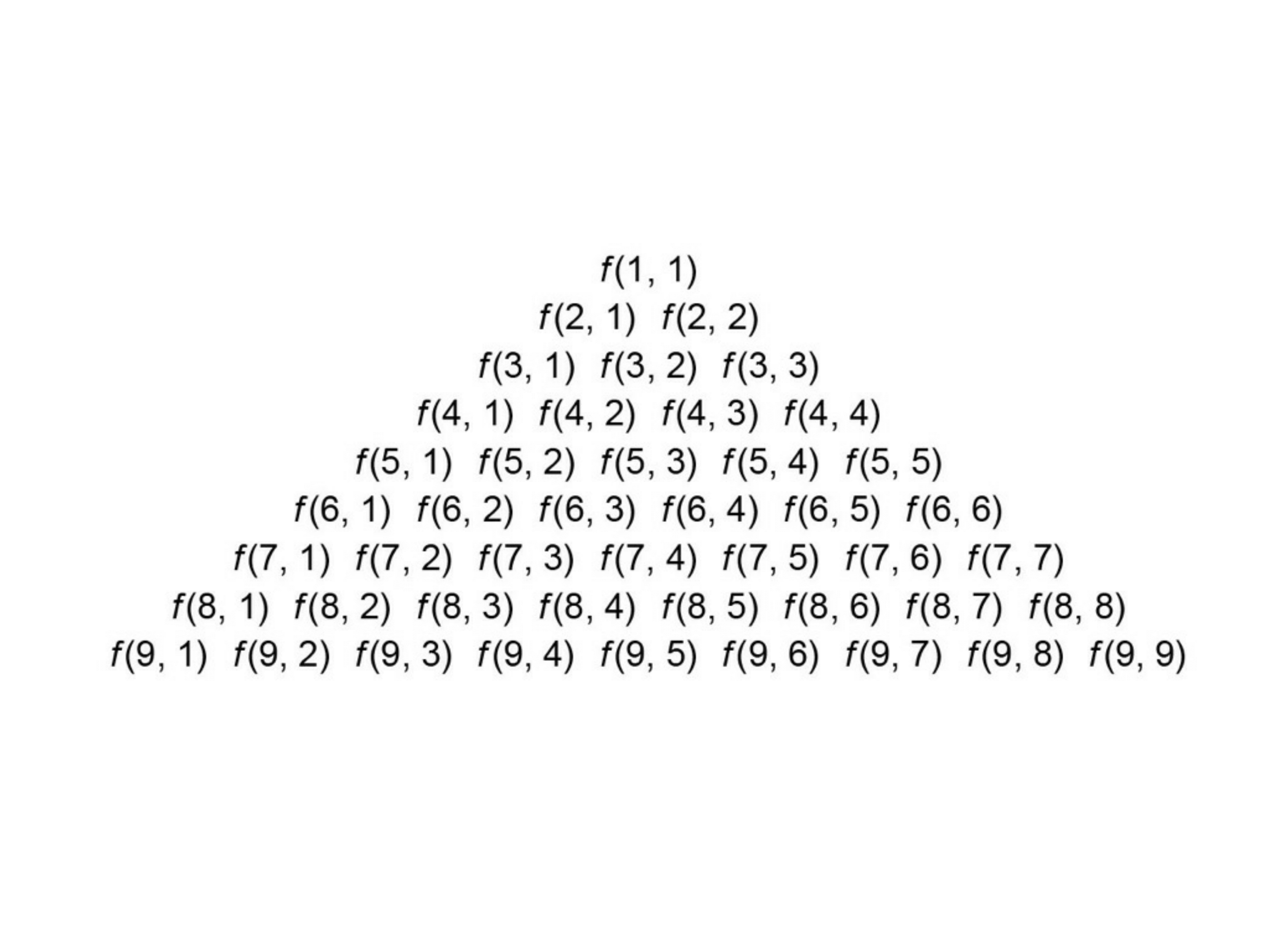When we construct this triangle, we use the method that is used for arranging nCm in Pascal's triangle.

When we calculate all the values of f(m,n), then we have the following triangle of fractions.

You will find that the structure of the triangle is similar to the Pascal's triangle, when you compare denominators and numerators.

For example, look at fractions in the blue triangle.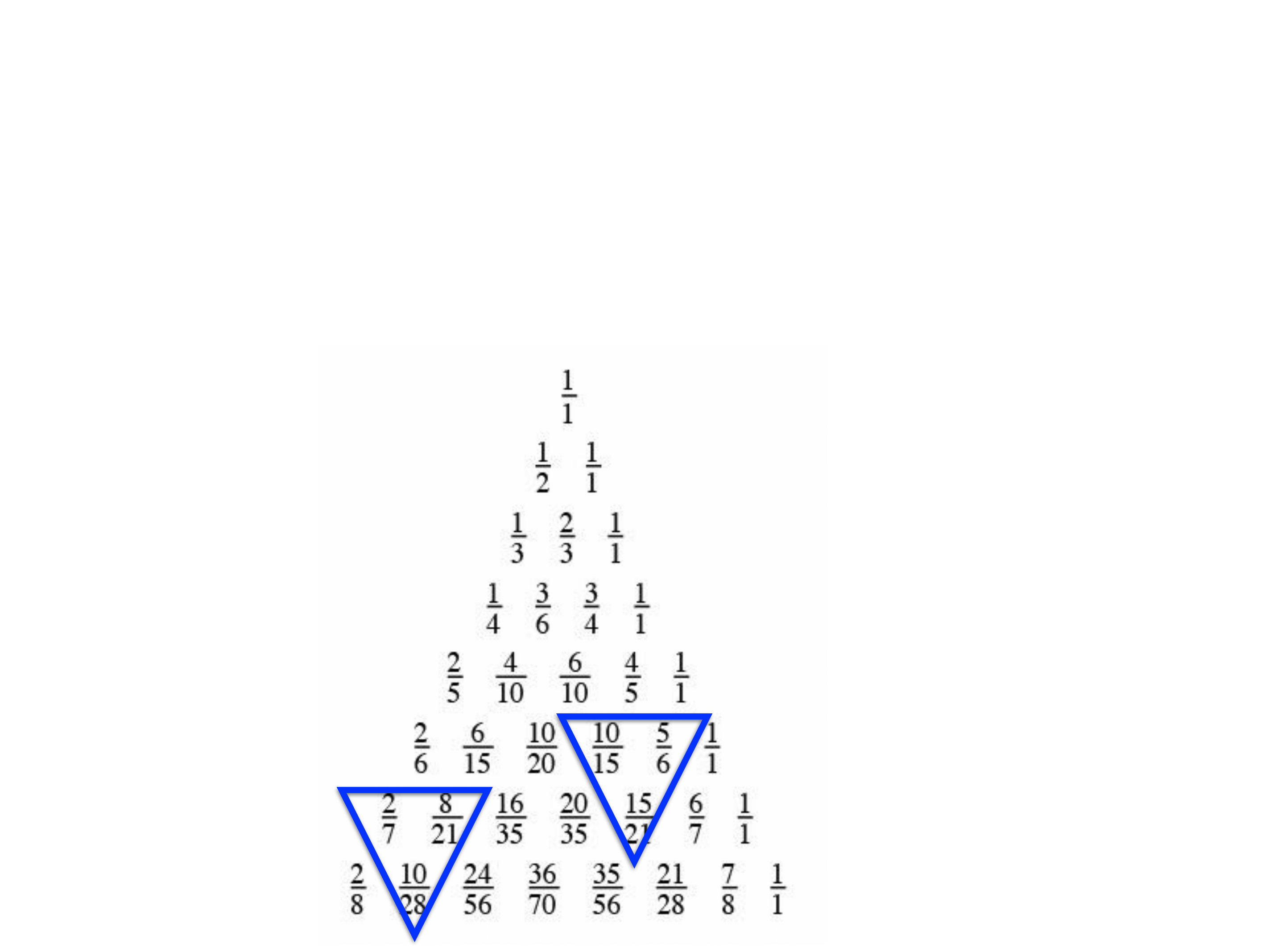The proof of the property which is similar to the Pascal's triangle is very simple.

Some teachers found this triangle of fractions interesting, and our article was published in "Osaka Mathematical education"

In 2007, some members of our research group changed numbers of players.

There are m red cards and n-m white cards are in a box, and p players take turns to draw a card from the box. Let f(p,n,m) be the probability of the first player's losing in the game.

By changing the values of n and m, we made the triangle below.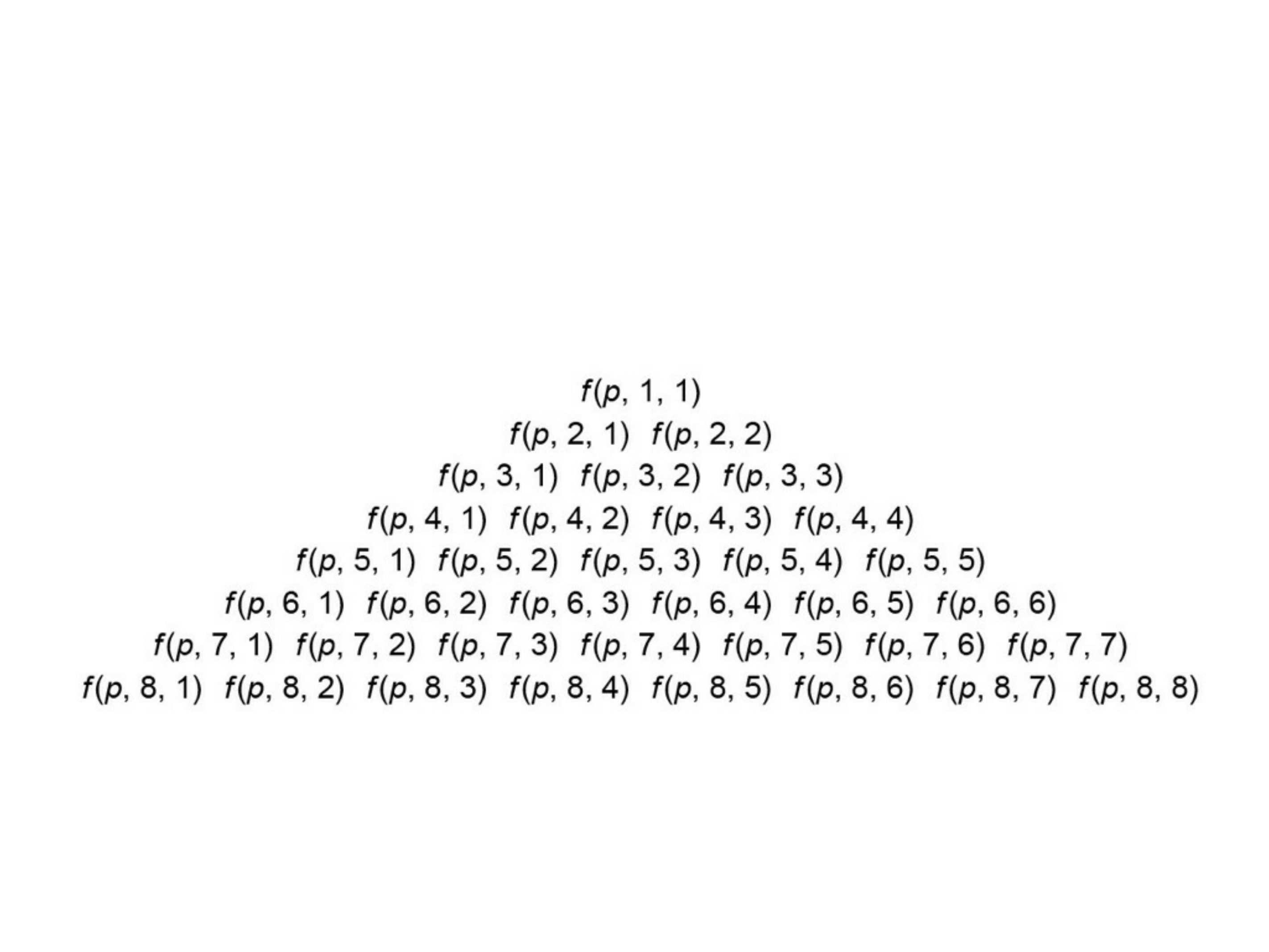The following is the calculation for p=4. You will find that this triangle is similar to the Pascal's triangle, when you compare denominator and numerator.For example, look at fractions in the blue triangle.

For an arbitrary natural number p, we proved the property which is similar to the Pascal's triangle. This result was published in Mathematical Gazette that is a well known math journal with more than 120 years of history.

### The Change of the Conditions of Losing the Game.

We had thought that there would not be any more thing to do about the research, but we were wrong.

There were a lot of things to do in this theme.

There are n red cards and n - m white cards in box. Two player A and B draw card alternately. If Player draws the red card, Player A loses. If Player B collects two red cards, Player B loses. The card will not be returned to the box.

The member of research team proposed this the modification on the condition in 2017.

The significant modification is that the condition of losing the game is different for A and B.

When we arranged the probabilities of the first player's losing in the game as a triangle, we discovered a very interesting fact.

Most parts of this triangle have the pascal-like property, but some parts do not.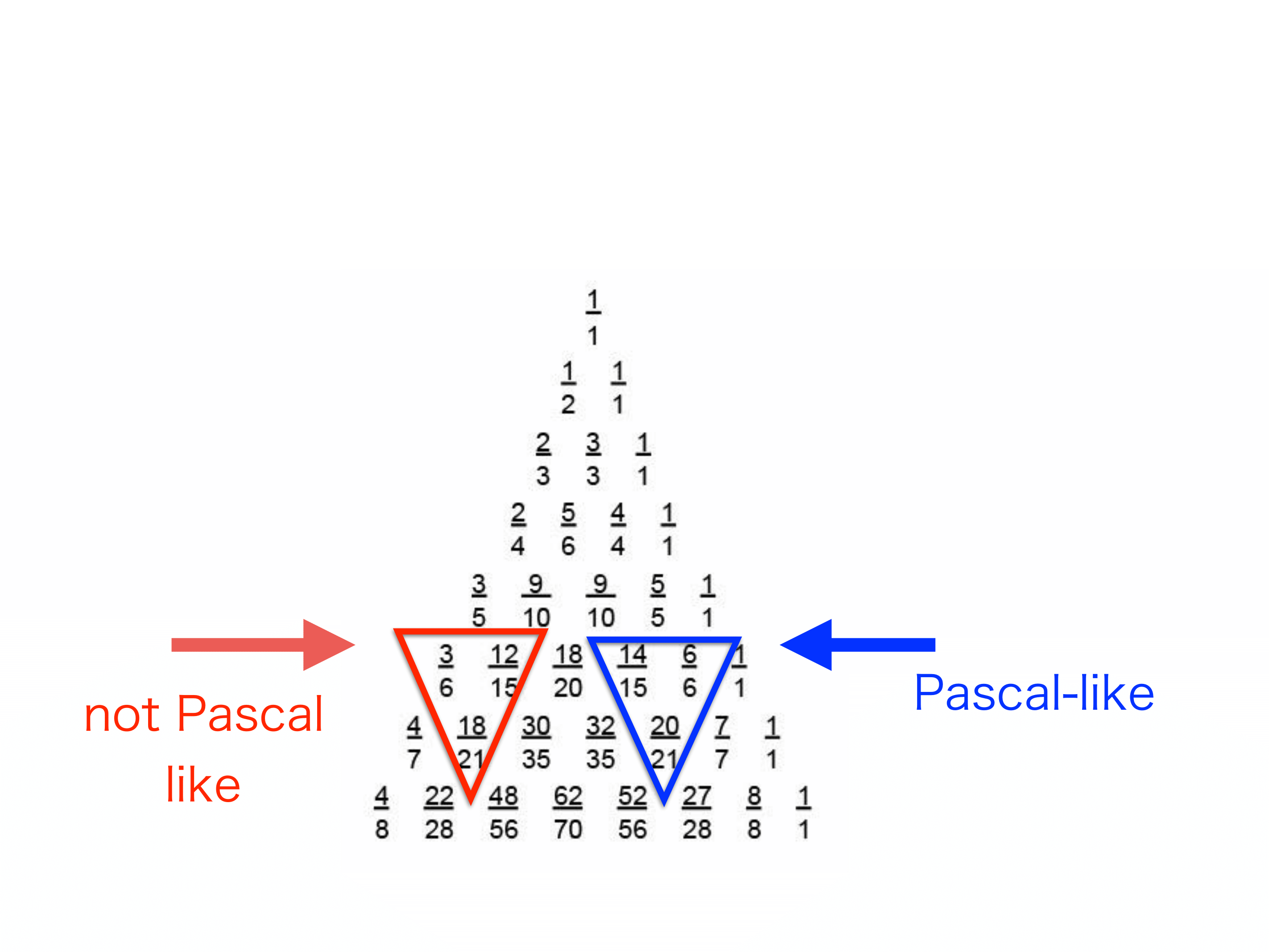For example, look at the fractions enclosed in red and blue triangle.

In most parts of this triangle of fractions, we have the property of Pascal's triangle, but there are some parts that do not have it, and the positions of these parts have a certain pattern.

We proved the existence of this pattern, and wrote a paper to be submitted to the conference "Japan Conference on Discrete and Computational Geometry, Graphs, and Games 2017", and it was accepted. We presented our research at the conference.

### Modifications on the Order of Drawing

There are still some parts that can be changed in the problem.

There are n red cards and n - m white cards in box. Two player draw card alternately. If a player draw the red card, the player loses. The card don't back into the box.

In 2018, we look for the order of drawing by which

the probabilities of losing is almost the same for A and B.

We discovered that the order ABBABAAB ...

is the best order under a certain condition.

We proved this, and wrote a paper to be submitted to the conference "Japan Conference on Discrete and Computational Geometry, Graphs, and Games 2017", and it was accepted. We presented our research at the conference.

Here, the order of + and − is as the followings.

(i)+, −, −, + (ii)+,−,−,+,−,+,+,−

(iii)+,−,−,+,−,+,+,−,−,+,+,−,+,−,−,+

### Mathematical Background

Here we study the mathematical background of this example.

Problem   There are one red card and five white cards in box. Two players take turns and draw card from the box. If one of players draw the red card, this player loses . Once a card is drawn, it will not be returned to the box.

Here, the order of drawing is A,B,A,B,A,B, and we call these the first, the second,...,the sixth round. It is clear that one of them loses in the game in these 6 rounds.

The probability of   A's losing in the first round is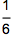. Next, we calculate the probability of   B's losing in the second round. First, we calculate the probability of A's drawing a white card in the first round that is 5/6. After that we calculate the probability of B's drawing a red card that is 1/5. Finally we multiply these two numbers, and we have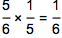.

A loses in the third round when A draws a white card, B draws a white card in the second round and A draws a red card in the third round. Then we have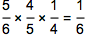. Similarly the probability of B's losing in the fourth round is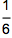. A loses in the 5th round with the probability of, and A loses in the 6th round with the probability.

Therefore the probability of A's losing in the game is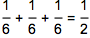, and the probability of B's losing in the game is.

Problem. Problem   There are m red cards and n-m white cards in box. Two players take turns and draw card from the box. If one of players draw the red card, this player loses . Once a card is drawn, it will not be returned to the box.

The game within n-m+1rounds. A loses in the first round with the probability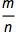, B loses in the second round with the probability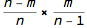, A loses in the third round with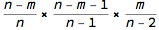,..., game ends in the y-th round with the probability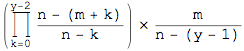.

Therefore the probability of A's losing in the game is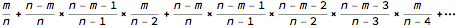, and maximal times of rounds for A is ⌊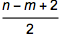⌋.

The game ends at the y-th round when there is not red cards among y-1 cards that are already drawn, and the y-th card that is drawn is red. The number of combination for this pattern of cards is n-yCm-1.

Then, we divide this by the number of all combinations,

and get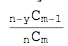In this way Player A’s losing in the game is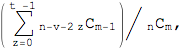where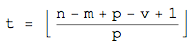The Pascal-like triangles presented in this example can be explained by the fact that the first player’s probability of losing in the game is calculated by combinations. As for the detailed explanation of the card game that produces this triangle, please read the paper written by our teacher and our group of students.

### Modification and Research

In this way you can make new problems just by changing numbers and rules of the original problem.

After that, you analyze the variant of the original problem. It is always better to use mathematical softwares, since students find it easier to do research with computer than do research only with a paper and pen.

Our research group has studied this problem since 1992. This group discovered new formulas in 1992, 2007,2017 and 2018. In recent years, the number of formulas that are discovered is increasing, as members of this group are beginning to understand the method of research.

To discover new formula sounds difficult, but it is not difficult to make new problem just by changing some parts of the original one. By this simple method, our research group has discovered a lot of new formulas and facts.

Reference

 Miyadera, R., Sakaguchi, M. and Masuda, M.:Mathematical theory of Russian roulette:Osaka Mathematics Education Journal,26 (1997).(in Japanese)

 Matsui, H., Minematsu, D., Yamauchi T., Miyadera, R.: Pascal-like triangles and Fibonacci-like sequences, Mathematical Gazette, (2010).

 Miyadera,R.,Suzuki,S., and etc., Generalized Pascal-Like Triangles, JCDCG^3 2018 (The 21th Japan Conference on Discrete and Computational Geometry, Graphs, and Games), Manila, August, 2018.

 Miyadera,R.,and etc., "Pascal-Like Triangles and Fibonacci-Like Sequences", JCDCG^3 2017, Tokyo, August, 2017.